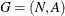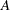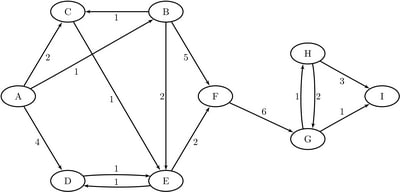### Graph Input Data

This section describes how to input a graph for analysis by PROC OPTNET. Letdefine a graph with a setof nodes and a setof links. There are two main methods for defining the set of linksas a SAS data set. The first is to use a list of links as described in the section Link Input Data. The second is to use an adjacency matrix as described in the section Adjacency Matrix Input Data.

To illustrate the different methods for input of a graph, consider the directed graph shown in Figure 2.5.

Figure 2.5: A Simple Directed GraphNotice that each node and link has associated attributes: a node label and a link weight.

#### Node Input Data

The DATA_NODES= option in the PROC OPTNET statement defines the data set that contains the list of nodes in the graph. This data set is used to assign node weights.

The nodes data set is expected to contain some combination of the following possible variables:

• `node`: the node label (this variable can be numeric or character)

• `weight`: the node weight (this variable must be numeric)

• `weight2`: the auxiliary node weight (this variable must be numeric)

You can specify any values you want for the data set variable names. If you use nonstandard names, you must identify the variables by using the DATA_NODES_VAR statement, as described in the section DATA_NODES_VAR Statement.

The data set that is specified in the DATA_LINKS= option defines the set of nodes that are incident to some link. If the graph contains a node that has no links (called a singleton node), then this node must be defined in the DATA_NODES data set. The following is an example of a graph with three links but four nodes, including a singleton node D:

```data NodeSetIn;
input label \$ @@;
datalines;
A B C D
;

input from \$ to \$ weight;
datalines;
A B 1
A C 2
B C 1
;
```

If you specify duplicate entries in the node data set, PROC OPTNET takes the first occurrence of the node and ignores the others. A warning is printed to the log.

#### Node Subset Input Data

For some algorithms you might want to process only a subset of the nodes in the input graph. You can accomplish this by using the DATA_NODES_SUB= option in the PROC OPTNET statement. You can use the node subset data set in conjunction with the SHORTPATH statement (see the section Shortest Path. The node subset data set is expected to contain some combination of the following variables:

• `node`: the node label (this variable can be numeric or character)

• `source`: whether to process this node as a source node in shortest path algorithms (this variable must be numeric)

• `sink`: whether to process this node as a sink node in shortest path algorithms (this variable must be numeric)

The values in the node subset data set determine how to process nodes when the SHORTPATH statement is processed. A value of 0 for the `source` variable designates that the node is not to be processed as a source; a value of 1 designates that the node is to be processed as a source. The same values can be used for the `sink` variable to designate whether the node is to be processed as a sink. The missing indicator (.) can also be used in place of 0 to designate that a node is not to be processed.

A representative example of a node subset data set that might be used with the graph in Figure 2.5 is as follows:

```data NodeSubSetIn;
input node \$ source sink;
datalines;
A 1 .
F . 1
E 1 .
;
```

The data set `NodeSubSetIn` indicates that you want to process the shortest paths from nodes A and E and the shortest paths to node F.